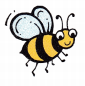# McGraw Hill Math Grade 1 Chapter 7 Lesson 8 Answer Key Solving Word Problems

All the solutions provided in McGraw Hill Math Grade 1 Answer Key PDF Chapter 7 Lesson 8 Solving Word Problems as per the latest syllabus guidelines.

## McGraw-Hill Math Grade 1 Answer Key Chapter 7 Lesson 8 Solving Word Problems

Solve

Add or subtract to solve. Write the sum or difference. Use objects to help.

Question 1.
There are 14 bees. 5 bees fly away. How many bees are left?
14 – 5 = _________9
Explanation:
Total number of bees are 14
Number of bees flew away = 5
14 – 5 = 9Question 2.
Ella draws 4 stars. She draws 2 more stars. How many stars does Ella draw?
4 + 2 = ___________4 + 2 = 6